## Saturday, 9 August 2014

### chapter 9 Methods for Evaluation of Limits 2

(C) RATIONALIZATION
In this method, the rationalization of an indeterminate expression leads to determinate one. The following examples elaborate this method.
(i)$\mathop {\lim }\limits_{x \to 0} \dfrac{{\sqrt {{x^2} + 1} - 1}}{{\sqrt {{x^2} + 16} - 4}}\left( {{\rm{of\, \,the\, \,indeterminate\,\, form \,\,}}\dfrac{{\rm{0}}}{{\rm{0}}}} \right)$
 Rationalizing both the numerator and the denominator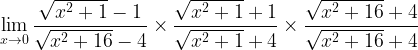$\mathop {\lim }\limits_{x \to 0} \dfrac{{\sqrt {{x^2} + 1} - 1}}{{\sqrt {{x^2} + 16} - 4}} \times \dfrac{{\sqrt {{x^2} + 1} + 1}}{{\sqrt {{x^2} + 1} + 4}} \times \dfrac{{\sqrt {{x^2} + 16} + 4}}{{\sqrt {{x^2} + 16} + 4}}$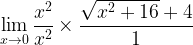$\mathop {\lim }\limits_{x \to 0} \dfrac{{{x^2}}}{{{x^2}}} \times \dfrac{{\sqrt {{x^2} + 16} + 4}}{1}$ A determinate form Cancelling out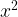${x^2}$ from the numerator and the denominator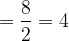$= \dfrac{8}{2} = 4$
(ii)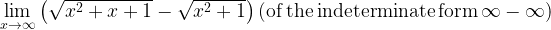$\mathop {\lim }\limits_{x \to \infty } \left( {\sqrt {{x^2} + x + 1} - \sqrt {{x^2} + 1} } \right) \left( {{\rm{of\, the \,indeterminate\, form \,}}\infty - \infty } \right)$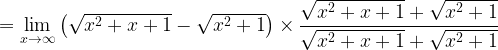$= \mathop {\lim }\limits_{x \to \infty } \left( {\sqrt {{x^2} + x + 1} - \sqrt {{x^2} + 1} } \right) \times \dfrac{{\sqrt {{x^2} + x + 1} + \sqrt {{x^2} + 1} }}{{\sqrt {{x^2} + x + 1} + \sqrt {{x^2} + 1} }}$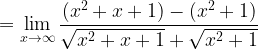$= \mathop {\lim }\limits_{x \to \infty } \dfrac{{({x^2} + x + 1) - ({x^2} + 1)}}{{\sqrt {{x^2} + x + 1} + \sqrt {{x^2} + 1} }}$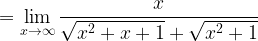$= \mathop {\lim }\limits_{x \to \infty } \dfrac{x}{{\sqrt {{x^2} + x + 1} + \sqrt {{x^2} + 1} }}$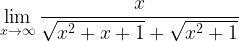$\mathop {\lim }\limits_{x \to \infty } \dfrac{x}{{\sqrt {{x^2} + x + 1} + \sqrt {{x^2} + 1} }}$ Divide the numerator and denominator by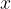$x$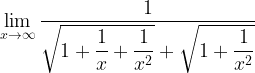$\mathop {\lim }\limits_{x \to \infty } \dfrac{1}{{\sqrt {1 + \dfrac{1}{x} + \dfrac{1}{{{x^2}}}} + \sqrt {1 + \dfrac{1}{{{x^2}}}} }}$
Rationalization has led us to another indeterminate form of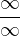$\dfrac{\infty }{\infty }$. However, it can easily be made determinate in the following manner:
Now as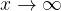$x \to \infty$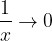$\dfrac{1}{x} \to 0$ and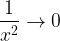$\dfrac{1}{{{x^2}}} \to 0$
Hence, the limit above reduces to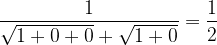$\dfrac{1}{{\sqrt {1 + 0 + 0} + \sqrt {1 + 0} }} = \dfrac{1}{2}$
(D) REDUCTION TO STANDARD FORMS
In this method, we try to reduce the given limit to one of the standard forms we studied earlier.
(i)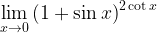$\mathop {\lim }\limits_{x \to 0} {\left( {1 + \sin x} \right)^{2\cot x}}$
This limit is of the indeterminate form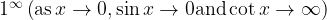${1^\infty }\left( {{\rm{as}}\,x \to 0,\sin x \to 0{\rm{and}}\cot x \to \infty } \right)$.
We proceed as follows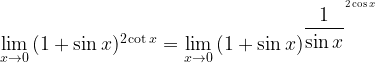$\mathop {\lim }\limits_{x \to 0} {(1 + \sin x)^{2\cot x}} = \mathop {\lim }\limits_{x \to 0} {(1 + \sin x)^{{{\dfrac{1}{{\sin x}}}^{^{2\cos x}}}}}$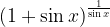${{{(1 + \sin x)}^{\frac{1}{{\sin x}}}}}$ This limit has the value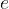$e$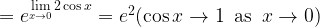$= {e^{\mathop {\lim }\limits_{x \to 0} 2\cos x}} = {e^2}(\cos x \to 1\,\,\,{\rm{as}}\,\,\,x \to 0)$
(ii)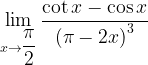$\mathop {\lim }\limits_{x \to \dfrac{\pi }{2}} \dfrac{{\cot x - \cos x}}{{{{(\pi - 2x)}^3}}}$
This limit is of the indeterminate form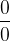$\dfrac{0}{0}$$\mathop {\lim }\limits_{x \to \dfrac{\pi }{2}} \dfrac{{\cot x - \cos x}}{{{{(\pi - 2x)}^3}}} = \mathop {\lim }\limits_{h \to 0} \dfrac{{\cot \left( {\dfrac{\pi }{2} + h} \right) - \cos \left( {\dfrac{\pi }{2} + h} \right)}}{{{{( - 2h)}^3}}}$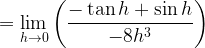$= \mathop {\lim }\limits_{h \to 0} \left( {\dfrac{{ - \tan h + \sin h}}{{ - 8{h^3}}}} \right)$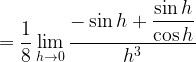$= \dfrac{1}{8}\mathop {\lim }\limits_{h \to 0} \dfrac{{ - \sin h + \dfrac{{\sin h}}{{\cos h}}}}{{{h^3}}}$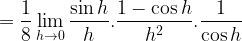$=\dfrac{1}{8}\mathop {\lim }\limits_{h \to 0} \dfrac{{\sin h}}{h}.\dfrac{{1 - \cos h}}{{{h^2}}}.\dfrac{1}{{\cos h}}$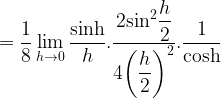$= \dfrac{1}{8}\mathop {\lim }\limits_{h \to 0} \dfrac{{\sinh }}{h}.\dfrac{{2{{\sin }^2}\dfrac{h}{2}}}{{4{{\left( {\dfrac{h}{2}} \right)}^2}}}.\dfrac{1}{{\cosh }}$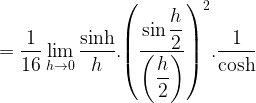$= \dfrac{1}{{16}}\mathop {\lim }\limits_{h \to 0} \dfrac{{\sinh }}{h}.{\left( {\dfrac{{\sin \dfrac{h}{2}}}{{\left( {\dfrac{h}{2}} \right)}}} \right)^2}.\dfrac{1}{{\cosh }}$
Let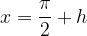$x = \dfrac{\pi }{2} + h$ so that as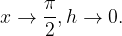$x \to \dfrac{\pi }{2},h \to 0.$
This expression now only contains the limits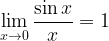$\mathop {\lim }\limits_{x \to 0} \dfrac{{\sin x}}{x} = 1$ and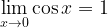$\mathop {\lim }\limits_{x \to 0} \cos x = 1$
Hence, the final result is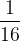$\dfrac{1}{{16}}$
We will now see examples based on the methods discussed above. We urge you to first try out all these examples on your own before viewing the solutions.